# CBSE Solutions for Class 10 Maths

#### Select CBSE Solutions for class 10 Subject & Chapters Wise :

Draw a line segment of length 6 cm. Using compasses and ruler, find a point P on it which divides it in the ratio 3 : 4. (2011D)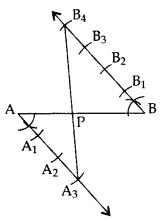=>  PA : PB = 3 : 4

Draw a line segment AB of length 7 cm. Using ruler and compasses, find a point P on AB such that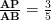. (2011OD)

AB = 7 cm, AB =… [Given in the question
AP : PB = 3 : 2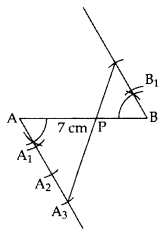AP : AB = 3 : 5

Constructions Class 10 Important Questions Short Answer-II (3 Marks)

Draw a triangle ABC in which AB = 5 cm, BC = 6 cm and ABC = 60°. Then construct a triangle whose sides aretimes the corresponding sides of ∆ABC. (2011D)

In ∆ABC
AB = 5 cm
BC = 6 cm
ABC = 60°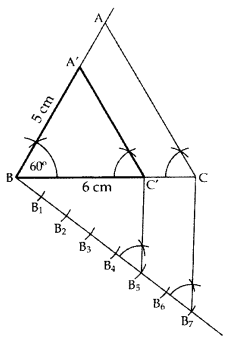∆A’BC’ is the required ∆.

Construct a triangle with sides 5 cm, 5.5 cm and 6.5 cm. Now construct another triangle, whose sides aretimes the corresponding sides of the given triangle. (20140D)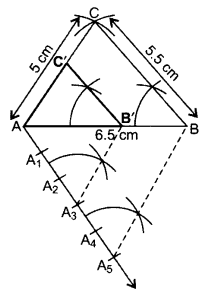∆AB’C’ is the required ∆.

Construct a right triangle in which the sides, (other than the hypotenuse) are of length 6 cm and 8 cm. Then construct another triangle, whose sides aretimes the corresponding sides of the given triangle. (2012D)

Here AB = 8 cm, BC = 6 cm and
Ratio =of corresponding sides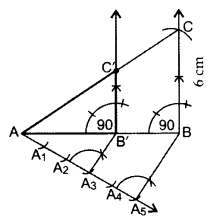∆AB’C’ is the required triangle.

Draw a triangle PQR such that PQ = 5 cm, P = 120° and PR = 6 cm. Construct another triangle whose sides aretimes the corresponding sides of ∆PQR. (2011D)

In ∆PQR,
PQ = 5 cm, PR = 6 cm,
P = 120°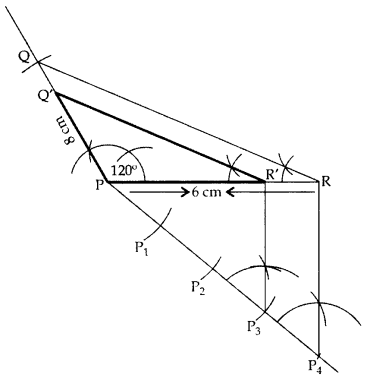∆POʻR’ is the required ∆.

Draw a triangle ABC with BC = 7 cm, B = 45° and C = 60°. Then construct another triangle, whose sides aretimes the corresponding sides of ∆ABC. (2012OD)

Here, BC = 7 cm, B = 45°, C = 60° and ratio istimes of corresponding sides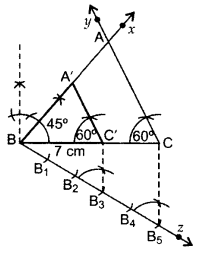∆A’BC’ is the required triangle.

Construct a triangle with sides 5 cm, 4 cm and 6 cm. Then construct another triangle whose sides aretimes the corresponding sides of first triangle. (2013D)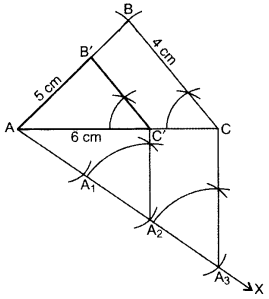Steps of Construction:

1. Draw ∆ABC with AC = 6 cm, AB = 5 cm, BC = 4 cm.
2. Draw ray AX making an acute angle with AÇ.
3. Locate 3 equal points A1, A2, A3 on AX.
4. Join CA3.
5. Join A2C’ || CA3.
6. From point C’ draw B’C’ || BC.

∆AB’C’ is the required triangle.

Draw a pair of tangents to a circle of radius 3 cm, which are inclined to each other at an angle of 60°. (2011OD)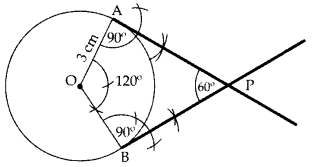PA & PB are the required tangents.

Draw a pair of tangents to a circle of radius 4.5 cm, which are inclined to each other at an angle of 45°. (2013OD)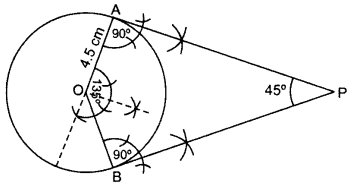Draw AOB = 135°, OAP = 90°, OBP = 90°

PA and PB are the required tangents.

Draw two tangents to a circle of radius 3.5 cm, from a point P at a distance of 6.2 cm from its centre. (2013OD)

OP = OC + CP = 3.5 + 2.7 = 6.2 cm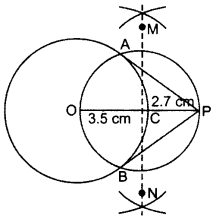AP & PB are the required tangents

Draw a right triangle ABC in which AB = 6 cm, BC = 8 cm and B = 90°. Draw BD perpendicular from B on AC and draw a circle passing through the points B, C and D. Construct tangents from A to this circle. (2014D, 2015OD)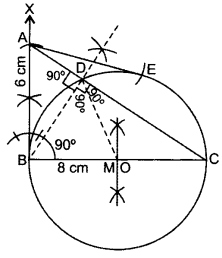Steps of Construction:

1. Draw BC = 8 cm.
2. From B draw an angle of 90°.
3. Draw an arc BA = 6 cm cutting the angle at A.
4. Join AC. ∆ABC is the required ∆.
5. Draw bisector of BC cutting BC at M.
6. Take Mas centre and BM as radius, draw a circle.
7. Take A as centre and AB as radius draw an arc cutting the circle at E. Join AE.

AB and AE are the required tangents.
Justification: ABC = 90° …[Given in the question
Since, OB is a radius of the circle.
AB is a tangent to the circle.
Also, AE is a tangent to the circle.

Find whether statement is True or False: - Tangent can be drawn without a circle

True

Find whether statement is True or False: - Tangent cannot be drawn without a circle

False

Find whether statement is True or False: - Angle between is radius and tangent is <90ᵒ

False

Find whether statement is True or False: - Angle between is radius and tangent is >90ᵒ

False

Find whether statement is True or False: - There are infinite tangent drawn from a circle.

True

Find whether statement is True or False: - There are finite tangent drawn from a circle

False

Find whether statement is True or False: - Angle between is radius and tangent is 90ᵒ

True

Draw a line segment AB of length 8 cm. Taking A as centre, draw a circle of radius 4 cm and taking B as centre, draw another circle of radius 3 cm. Construct tangents to each circle from the centre of the other circle. (2014OD)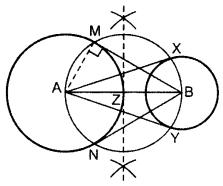Draw two circles on A and B as asked.
Z is the mid-point of AB.
From Z, draw a circle taking ZA = ZB as radius,
so that the circle intersects the bigger circle at M and N and smaller circle at X and Y.
Join AX and AY, BM and BN.
BM, BN are the reqd. tangents from external point B.
AX, AY are the reqd. tangents from external point A.

Draw a triangle ABC with side BC = 7 cm, B = 45° and ∆A = 105°. Then construct a triangle whose sides are 3/5 times the corresponding sides of ∆ABC. (2011D)

In ∆ABC, A + B + C = 180° … [angle sum property of a ∆
105° + 45° + C = 180°

C = 180° – 105° – 45o = 30°
BC = 7 cm∆A’BC’ is the required ∆.

Draw a triangle ABC with side BC = 6 cm, C = 30° and A = 105°. Then construct another triangle whose sides aretimes the corresponding sides of ∆ABC. (2012D)

BC = 6 cm, A = 105° and C = 30°In ∆ABC,
A + B + C = 180° …[Angle-sum-property
105° +
B + 30o = 180°
B = 180° – 105° – 30o = 45°
=>  ∆A’BC’ is the required ∆.

Draw a triangle with sides 5 cm, 6 cm and 7 cm. Then construct another triangle whose sides are 2/3 times the corresponding sides of the first triangle. (2012OD)

Given AB = 5 cm, BC = 7 cm, AC = 6 cm and ratio is  2/3 of corresponding sides.=>  ∆A’BC’ is the required triangle.

Construct an isosceles triangle whose base is 6 cm and altitude 4 cm. Then construct another triangle whose sides are ¾  times the corresponding sides of the isosceles triangle. (2015D)=>  ∆A’BC’ is the required triangle.

Draw a line segment AB of length 7 cm. Taking A as centre, draw a circle of radius 3 cm and taking B as centre, draw another circle of radius 2 cm. Construct tangents to each circle from the centre of the other circle. (2015D)Draw two circles on A and B.
Z is the mid-point of AB.
From Z, draw a circle taking ZA = ZB as radius,
such that the circle intersects the bigger circle at M & N and smaller circle at X & Y.
Join AX & AY, BM & BN.
BM, BN are the required tangents from external point B.
AX, AY are the required tangents from external point A.
Justification:

AMB = 90° …[Angle of a semi-circle

AM is a radius of the given circle.
=> BM is a tangent to the circle
Similarly, BN, AX and AY are also tangents.1 OC A 2.7cm 2 CP B 6.2cm 3 OP C 3.5cm 4 ∠OAP D 90ᵒ

1-C, 2-A, 3-B, 4-D1 OA A 135ᵒ 2 ∠OAP B 4.5 cm 3 ∠AOB C 45ᵒ 4 ∠APB D 90ᵒ

1-B, 2-D, 3-A, 4-C1 AP: BP A 7cm 2 ∠A B 2.8cm 3 BP C ∠B 4 AB D 3:2

1-D, 2-C, 3-B, 4-A1 AP: BP A ∠A3PA 2 ∠A B 3:4 3 AA1 C ∠B 4 ∠B4PB D B1B

1-B, 2-C, 3-D, 4-A1 BC:BC’ A 5:2 2 ∠B B 5cm 3 BC’:CC’ C 60ᵒ 4 AB D 7:5

1-D, 2-C, 3-A, 4-B

### Chapter 11 : Constructions

11th chapter of Maths is all about construction of line segments, division of a Line Segment and Construction of a Circle, Constructions of Tangents to a circle using analytical approach. Also students have to provide justification of each answer. Some of the ideas applied in this chapter:
1. The locus of a point that moves in an identical distance from 2 points, is normal to the line joining both the points.
2. Perpendicular or Normal means right angles whereas, bisector cuts a line segment in two half.
3. The design of different shapes utilizing a pair of compasses and straightedge or ruler.

### Browse & Download CBSE Books For Class 10 - All Subjects

The GSEB Books for class 10 are designed as per the syllabus followed Gujarat Secondary and Higher Secondary Education Board provides key detailed, and a through solutions to all the questions relating to the GSEB textbooks.

The purpose is to provide help to the students with their homework, preparing for the examinations and personal learning. These books are very helpful for the preparation of examination.

For more details about the GSEB books for Class 10, you can access the PDF which is as in the above given links for the same.